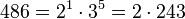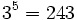# Groups of order 486

## Contents

See pages on algebraic structures of order 486| See pages on groups of a particular order

## Statistics at a glance

The number 486 has prime factors 2 and 3. The prime factorization is:$\! 486 = 2^1 \cdot 3^5= 2 \cdot 243$

There are only two prime factors of this number. Order has only two prime factors implies solvable (by Burnside's$p^aq^b$-theorem) and hence all groups of this order are solvable groups (specifically, finite solvable groups). Another way of putting this is that the order is a solvability-forcing number. In particular, there is no simple non-abelian group of this order.

The 3-Sylow subgroup is a group of order$3^5 = 243$ and is a normal Sylow subgroup. The 2-Sylow subgroup is isomorphic to cyclic group:Z2 and the whole group is an internal semidirect product of the 3-Sylow subgroup and the 2-Sylow subgroup. There are two possibilities:

• The group is a finite nilpotent group: In this case, it is a direct product of its 3-Sylow subgroup and its 2-Sylow subgroup.
• The group is the semidirect product of its 3-Sylow subgroup by a subgroup of orer two generated by a non-identity automorphism.

## GAP implementation

The order 486 is part of GAP's SmallGroup library. Hence, any group of order 486 can be constructed using the SmallGroup function by specifying its group ID. Also, IdGroup is available, so the group ID of any group of this order can be queried.

Further, the collection of all groups of order 486 can be accessed as a list using GAP's AllSmallGroups function.

Here is GAP's summary information about how it stores groups of this order, accessed using GAP's SmallGroupsInformation function:

gap> SmallGroupsInformation(486);

There are 261 groups of order 486.
They are sorted by their Frattini factors.
1 has Frattini factor [ 6, 1 ].
2 has Frattini factor [ 6, 2 ].
3 - 41 have Frattini factor [ 18, 3 ].
42 - 61 have Frattini factor [ 18, 4 ].
62 - 90 have Frattini factor [ 18, 5 ].
91 - 132 have Frattini factor [ 54, 12 ].
133 - 179 have Frattini factor [ 54, 13 ].
180 - 189 have Frattini factor [ 54, 14 ].
190 - 219 have Frattini factor [ 54, 15 ].
220 - 226 have Frattini factor [ 162, 51 ].
227 - 239 have Frattini factor [ 162, 52 ].
240 - 246 have Frattini factor [ 162, 53 ].
247 - 249 have Frattini factor [ 162, 54 ].
250 - 255 have Frattini factor [ 162, 55 ].
256 - 261 have trivial Frattini subgroup.

For the selection functions the values of the following attributes
are precomputed and stored:
IsAbelian, IsNilpotentGroup, IsSupersolvableGroup, IsSolvableGroup,
LGLength, FrattinifactorSize and FrattinifactorId.

This size belongs to layer 2 of the SmallGroups library.
IdSmallGroup is available for this size.